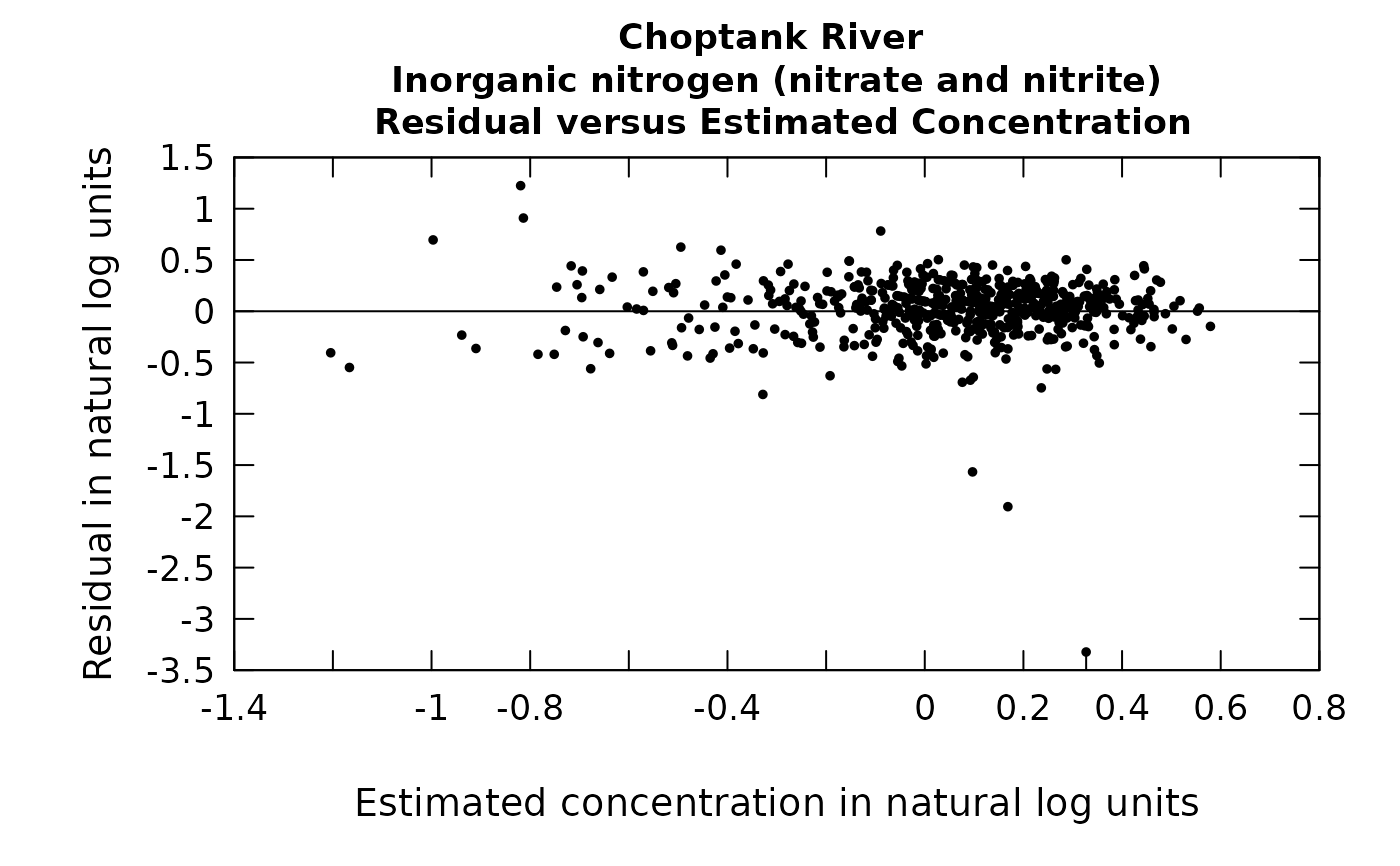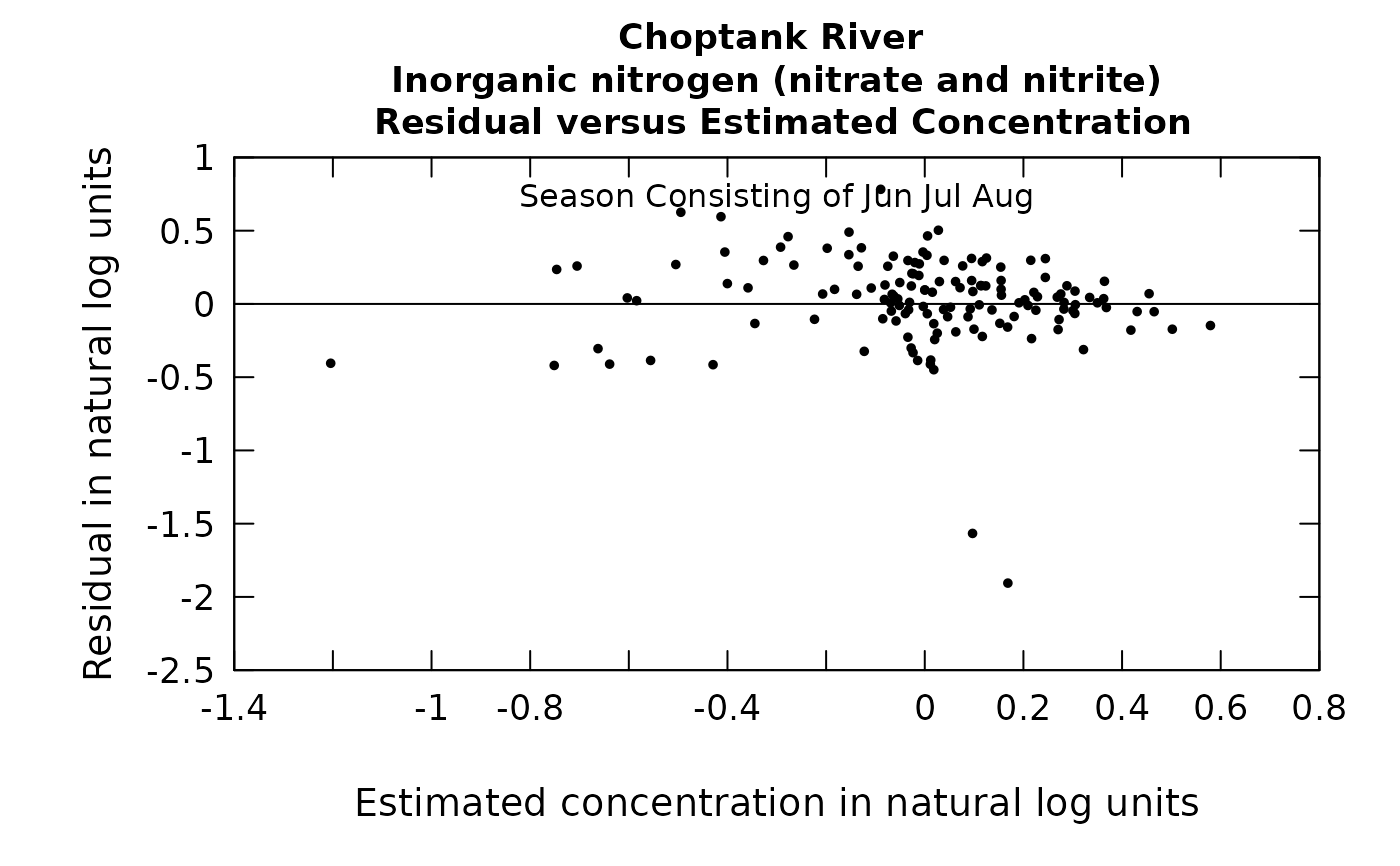This function produces a plot of the residuals from WRTDS, expressed in natural log concentration units versus the estimated values, also in natural log concentration units. These estimates are the log-space estimates prior to bias-correction. The function provides an alternative for viewing the standardized residuals, where the each residual is divided by its estimated standard error.

Although there are a lot of optional arguments to this function, most are set to a logical default.

Data come from named list, which contains a Sample dataframe with the sample data, and an INFO dataframe with metadata.

## Usage

plotResidPred(eList, stdResid = FALSE, tinyPlot = FALSE,
printTitle = TRUE, col = "black", lwd = 1, cex = 0.8,
cex.axis = 1.1, cex.main = 1.1, customPar = FALSE,
randomCensored = FALSE, concLab = 1, ...)

## Arguments

eList

named list with at least the Sample and INFO dataframes

stdResid

logical variable, if TRUE it uses the standardized residual, if FALSE it uses the actual, default is FALSE

tinyPlot

logical variable, if TRUE plot is designed to be plotted small as part of a multipart figure, default is FALSE.

printTitle

logical variable if TRUE title is printed, if FALSE not printed (this is best for a multi-plot figure)

col

color of points on plot, see ?par 'Color Specification'

lwd

number line width

cex

numerical value giving the amount by which plotting symbols should be magnified

cex.axis

magnification to be used for x and y labels relative to the current setting of cex

cex.main

magnification to be used for main titles relative to the current setting of cex

customPar

logical defaults to FALSE. If TRUE, par() should be set by user before calling this function (for example, adjusting margins with par(mar=c(5,5,5,5))). If customPar FALSE, EGRET chooses the best margins depending on tinyPlot.

randomCensored

logical. Show censored residuals as randomized.

concLab

object of concUnit class, or numeric represented the short code, or character representing the descriptive name. By default, this argument sets concentration labels to use either Concentration or Conc (for tiny plots). Units are taken from the eList$INFO$param.units. To use any other words than "Concentration" see vignette(topic = "units", package = "EGRET").

...

arbitrary graphical parameters that will be passed to genericEGRETDotPlot function (see ?par for options)

selectDays, genericEGRETDotPlot

## Examples

eList <- Choptank_eList
# Water year:
plotResidPred(eList)# Graphs consisting of Jun-Aug
eList <- setPA(eList, paStart=6,paLong=3)
plotResidPred(eList)